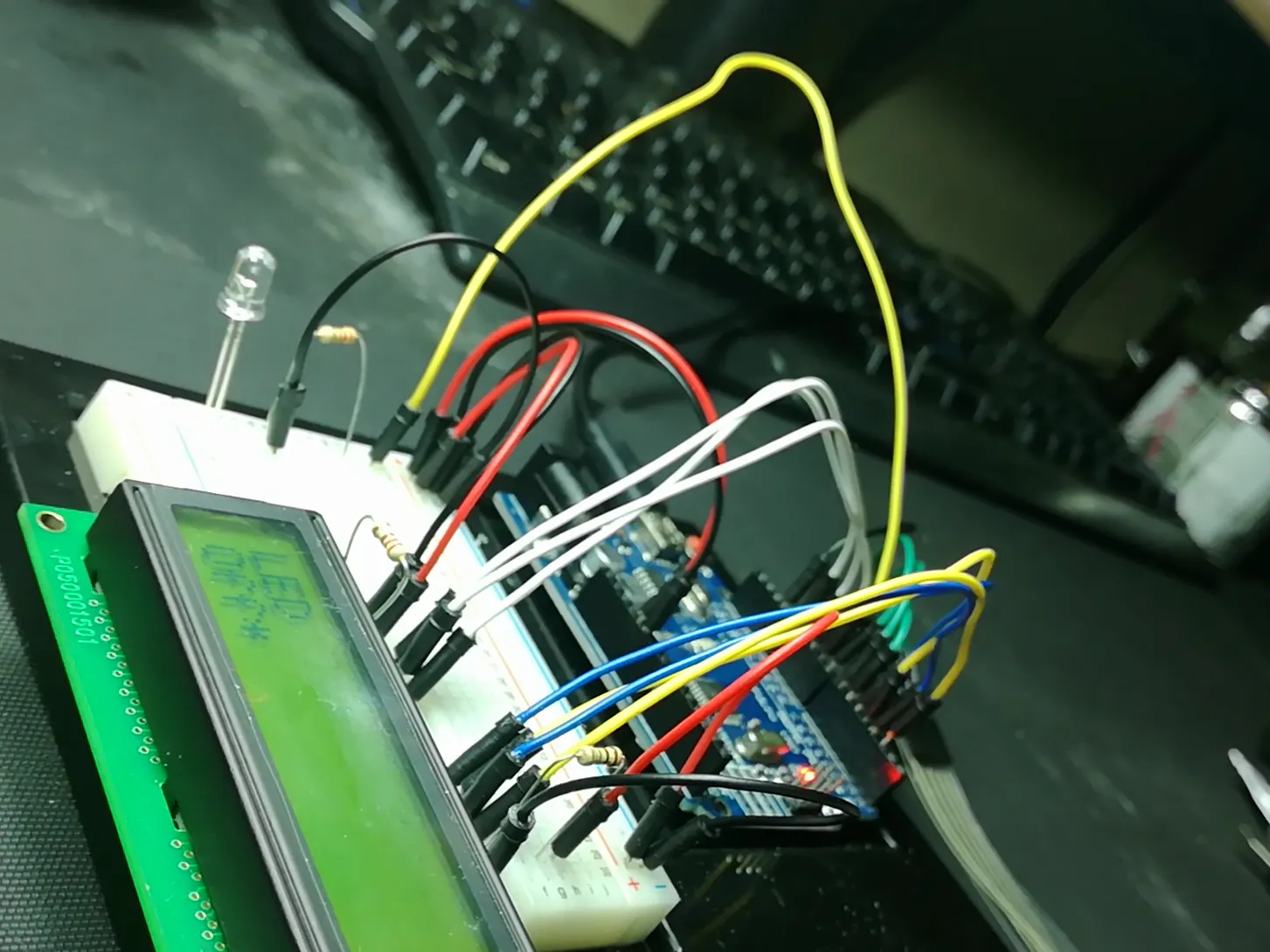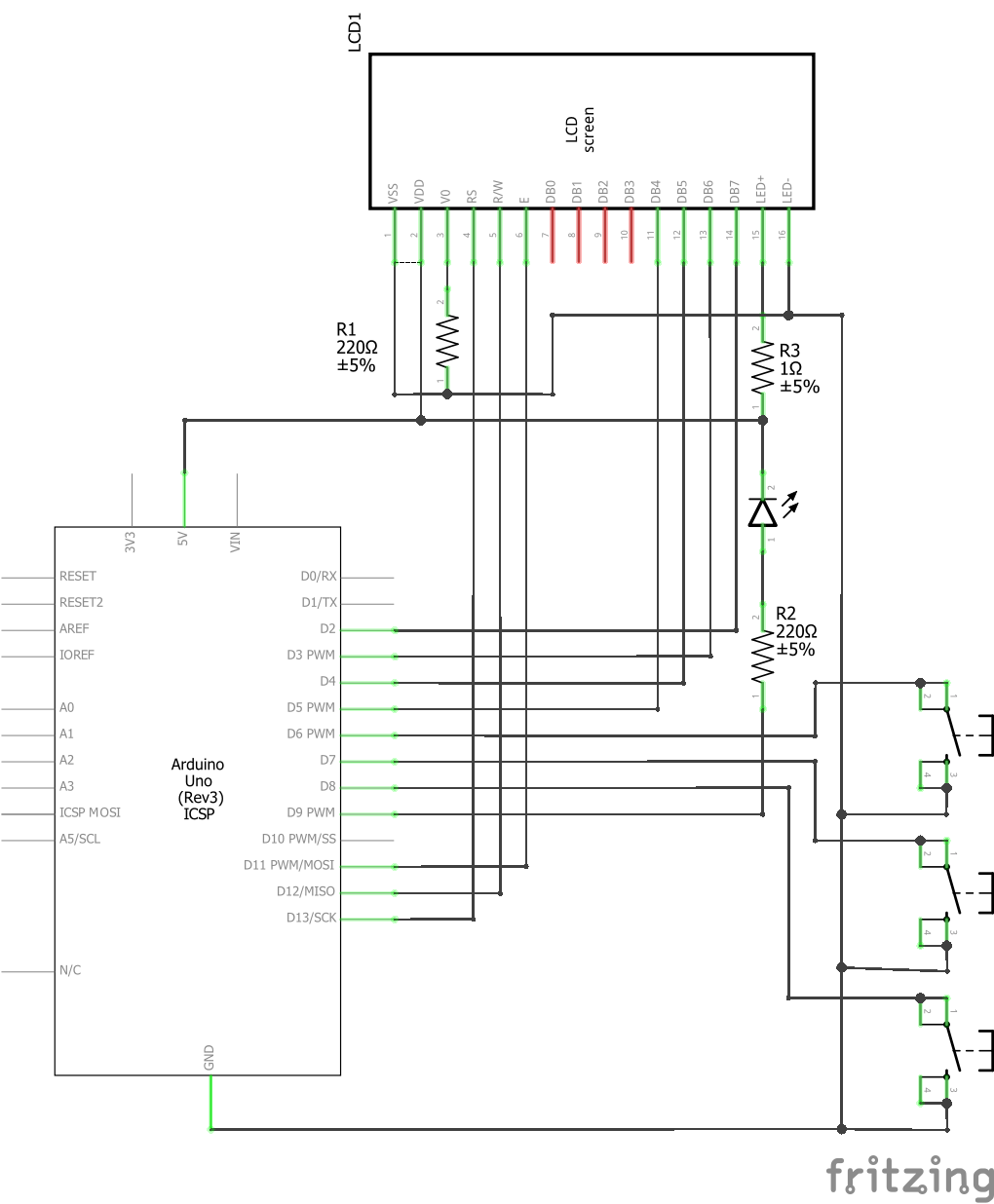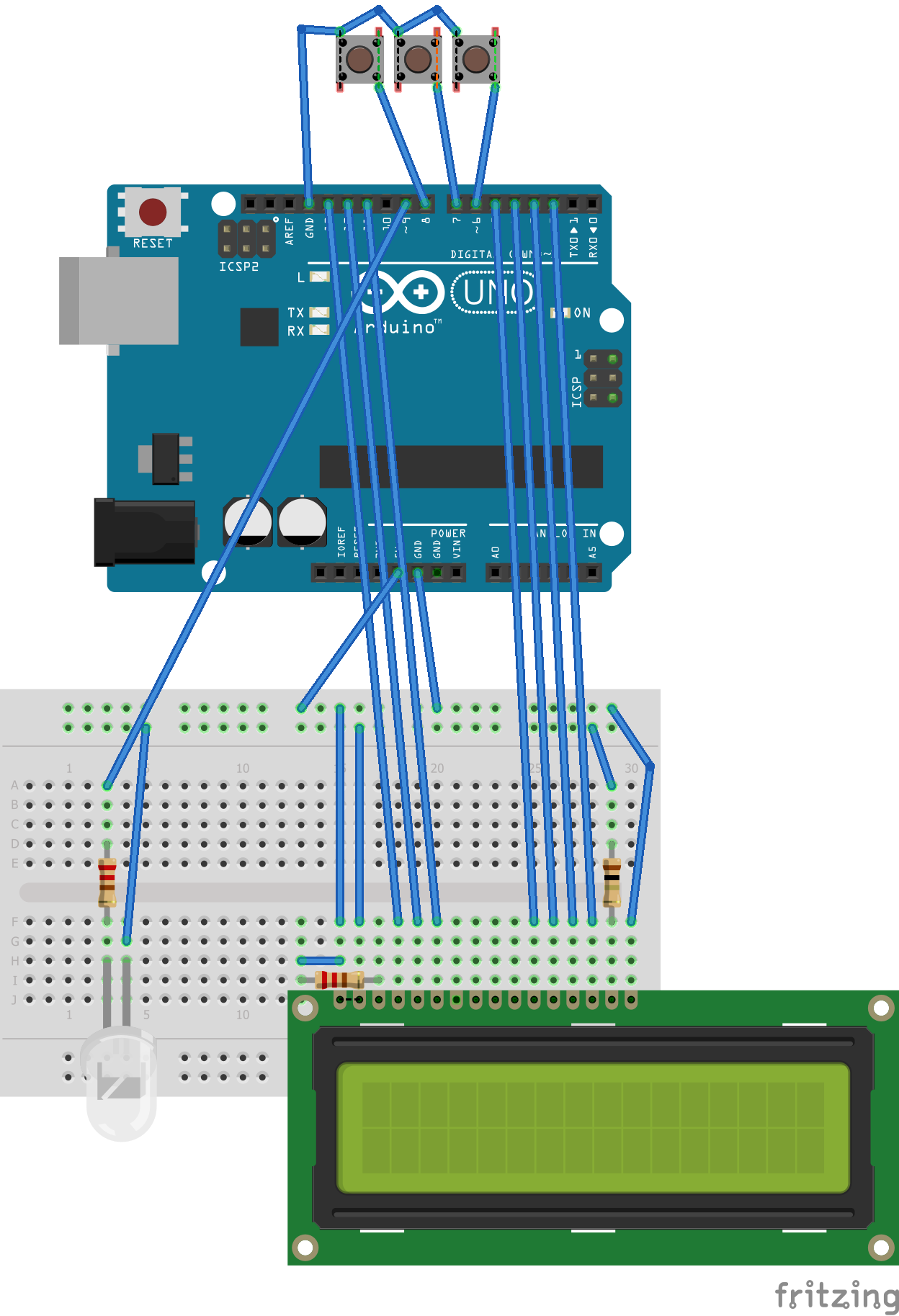I've created a simple menu with an LCD that controls what an LED does. The menu is easy to edit and adapt for various projects.

• 139,506 views
• 60 respects

Components and supplies

Today we are hooking up a LCD screen and controlling a LED all with a simple Menu program.

We will need the following:
• Some Jumper wires
• Some Buttons (I'm using a 4 by 1 Button Membrane)
• 1 x LCD Screen, (I'm using the LMB 162ABC 5v LCD)
• 2 x 220 ohm resistors
• 1 x 1 ohm resistor
• 1 x LED
• An Arduino
if (typeof(lightBoxImages) == 'undefined') { lightBoxImages = {}; } lightBoxImages['08800d05ce'] = [{ URL: 'https://hackster.imgix.net/uploads/attachments/364430/parts_nuly1cSDO3.png?auto=compress%2Cformat&w=1280&h=960&fit=max', caption: '', type: 'image' }];

As I always do, I connect ground from the Arduino to negative Rail and connect 5v of the Arduino to the 5v rail.

The LMB162ABC has 16 pins. Pin 1 and 16 are numbered.

• Place the LCD onto the Breadboard. (You might need to solder header pins onto your LCD screen first)
• Place a lead from the Ground rail to pin 1 of the LCD
• Connect pin 2 of the LCD to the Positive rail of the breadboard
• Place 1 x 220 Ohm resistor between pin 3 and 1 of the LCD screen (This controls the Contrast of the screen)
if (typeof(lightBoxImages) == 'undefined') { lightBoxImages = {}; } lightBoxImages['7a466f754c'] = [{ URL: 'https://hackster.imgix.net/uploads/attachments/364436/lcd_power_nAQLD4whkh.png?auto=compress%2Cformat&w=1280&h=960&fit=max', caption: '', type: 'image' }];
• Connect pins 10, 11 and 12 of the Arduino to pins 6, 5 and 4 of the LCD (Pins 4, 5 and 6 on the LCD are RS, R/W and Enable for the LMB162ABC)
if (typeof(lightBoxImages) == 'undefined') { lightBoxImages = {}; } lightBoxImages['eb82459ab2'] = [{ URL: 'https://hackster.imgix.net/uploads/attachments/364444/lcd_control_k7LwaOGZYu.png?auto=compress%2Cformat&w=1280&h=960&fit=max', caption: '', type: 'image' }];
• Connect pins 2, 3, 4 and 5 of the Arduino to pins 14, 13, 12 and 11 of the LCD (These are the Data pins of the LCD)
if (typeof(lightBoxImages) == 'undefined') { lightBoxImages = {}; } lightBoxImages['a15db65bf3'] = [{ URL: 'https://hackster.imgix.net/uploads/attachments/364435/lcd_data_dkO4FshZ6v.png?auto=compress%2Cformat&w=1280&h=960&fit=max', caption: '', type: 'image' }];
• Connect pin 16 to Ground
• Connect a 1ohm Resistor from the Positive rail to a terminal strip
• (I used an online calculator to get the value of the resistor using the figures from the LCD datasheet)
• Connect pin 15 of the LCD to the terminal strip with the 1 ohm resistor (Pin 15 and pin 16 of the LCD control the backlight.)
if (typeof(lightBoxImages) == 'undefined') { lightBoxImages = {}; } lightBoxImages['aa0f440348'] = [{ URL: 'https://hackster.imgix.net/uploads/attachments/364437/lcd_backlight_7acbzUSkO5.png?auto=compress%2Cformat&w=1280&h=960&fit=max', caption: '', type: 'image' }];

Next section we will tackle is the one LED
• Place the led between 2 terminal strips
• Place a resistor from the Anode to another Terminal Strip
• Connect pin 9 of the Arduino to the Resistor
• Connect the Cathode of the LED to a negative rail
if (typeof(lightBoxImages) == 'undefined') { lightBoxImages = {}; } lightBoxImages['ca32f555f4'] = [{ URL: 'https://hackster.imgix.net/uploads/attachments/364439/led_mfsH0T8T4b.png?auto=compress%2Cformat&w=1280&h=960&fit=max', caption: '', type: 'image' }];

Let's connect the 4 button Membrane

The pins 1, 2, 3 , 4 and 5 on the membrane that I have, correspond to buttons 2, 1, 4, 3 and Common. I am only going to use 3 of the buttons:

• Connect a lead from the common pin of the membrane to the Ground of the Arduino
• Connect 3 other leads from the membrane to pins 6, 7 and 8 of the Arduino
if (typeof(lightBoxImages) == 'undefined') { lightBoxImages = {}; } lightBoxImages['f897a9dd81'] = [{ URL: 'https://hackster.imgix.net/uploads/attachments/364440/buttons_50axMfi4us.png?auto=compress%2Cformat&w=1280&h=960&fit=max', caption: '', type: 'image' }];

The Code

I designed this menu program to easily edit and execute code where required. When a button is pressed it will either Navigate the Menu or Execute Code.

Execute code

• In this example the executed part will control the LED
• Turning the LED: ON, OFF, fading the LED or blinking the LED

In the Navigate Section

• It figures out where you are in the menu
• Finds possible places you can navigate to
• Then allows you to choose where you can navigate to, using the buttons
if (typeof(lightBoxImages) == 'undefined') { lightBoxImages = {}; } lightBoxImages['6328f64af3'] = [{ URL: 'https://hackster.imgix.net/uploads/attachments/364438/rect4185_gKg80BUDAC.png?auto=compress%2Cformat&w=1280&h=960&fit=max', caption: '', type: 'image' }];

Instead of the numbers on the button membrane I stuck masking tape labels to assist with navigation.

if (typeof(lightBoxImages) == 'undefined') { lightBoxImages = {}; } lightBoxImages['2e357ef8c5'] = [{ URL: 'https://hackster.imgix.net/uploads/attachments/364443/painters_tape_8M2SBWFpAi.png?auto=compress%2Cformat&w=1280&h=960&fit=max', caption: '', type: 'image' }];

Code

The Arduino IDE code to run the Arduino Uno
#include <LiquidCrystal.h>
LiquidCrystal lcd(12, 11, 10, 5, 4, 3, 2); // ARDUINO UNO
//LiquidCrystal lcd(3, 2, 1, 7, 8, 9, 10); // ATTINY84
byte buttons[] = {6,7,8}; //10 for additional pin
const byte nrButtons = 3; // change to 4 if additional button added
};

int t;
byte pressedButton, currentPos,currentPosParent, possiblePos, possiblePosCount, possiblePosScroll = 0;
String parent = "";

int brightness;
int LCDtoggle = 0;
unsigned long timer = (millis() / 10);
int ledMode = 0;

possiblePosCount = 0;
while (possiblePosCount == 0) {

for (t = 1; t < menusize;t++) {
possiblePos[possiblePosCount]  =  t;
possiblePosCount = possiblePosCount + 1;
}
}

//find the current parent for the current menu
currentPosParent = 0;
for (t = 0; t < menusize; t++) {
if (parent == menu[t]) {currentPosParent = t;}
}

// reached the end of the Menu line
if (possiblePosCount == 0) {
switch (currentPos) {
case 2:
case 3:
case 4:
case 5://Choose between 2,3,4,5
for (t = 2; t<6; t++) {
}
}
break;
case 6: //Toggle
} else {
}

break;
}

//Set Variables
switch (currentPos) {
case 2:
ledMode = 0;
break;
case 3:
ledMode = 1;
break;
case 4:
brightness = 128;
ledMode = 2;
break;
case 5:
brightness = 0;
ledMode = 3;
break;
case 6:
LCDtoggle = abs(LCDtoggle - 1);
digitalWrite(13,LCDtoggle);
break;
}

// go to the parent
currentPos = currentPosParent;
}
}
lcd.clear();

}

// Look for a button press
byte checkButtonPress() {
byte bP = 0;
byte rBp = 0;
for (t = 0; t<nrButtons;t++) {
if (digitalRead(buttons[t]) == 0) {bP = (t + 1);}
}
rBp = bP;
while (bP != 0) { // wait while the button is still down
bP = 0;
for (t = 0; t<nrButtons;t++) {
if (digitalRead(buttons[t]) == 0) {bP = (t + 1);}
}
}
return rBp;
}

void setup() {

lcd.begin(16,2);
lcd.clear();

for (t=0;t<nrButtons;t++) {
pinMode(buttons[t],INPUT_PULLUP);
}

pinMode(13,OUTPUT);
pinMode(9,OUTPUT);

lcd.setCursor(0,0); lcd.print("HELLO");
delay(1000);

}

void loop() {

switch (ledMode) {
case 0:
analogWrite(9, 0);
break;
case 1:
analogWrite(9, 255);
break;
case 3:
if ((millis() / 10) - timer > 30) {
timer = (millis() / 10);
brightness = abs(brightness - 255);
timer = (millis() / 10);
analogWrite(9, brightness);
}
break;
case 2:
if ((millis() / 10) - timer > 3) {
timer = (millis() / 10);
analogWrite(9, brightness);
if (brightness <= 0 || brightness >= 255) {
}
}
break;
}

pressedButton = checkButtonPress();
if (pressedButton !=0) {
switch (pressedButton) {
case 1:
possiblePosScroll = (possiblePosScroll + 1) % possiblePosCount; // Scroll
break;
// If I wanted a 4 button controll of the menu
//case 4:
//  possiblePosScroll = (possiblePosScroll + possiblePosCount - 1) % possiblePosCount; // Scroll
//break;
case 2:
currentPos = possiblePos[possiblePosScroll]; //Okay
break;
case 3:
currentPos = currentPosParent; //Back
possiblePosScroll = 0;
break;
}
}
}
functionsArduino
Supporting Functions
String mid(String str, int start, int len) {
int t = 0;
String u = "";
for (t = 0; t < len;t++) {
u = u + str.charAt(t+start-1);
}
return u;
}

int inStrRev(String str,String chr) {
int t = str.length()-1;
int u = 0;
while (t>-1) {
if (str.charAt(t)==chr.charAt(0)) {
u = t+1;t = -1;
}
t = t - 1;
}
return u;
}

int len(String str) {
return str.length();
}

SchematicsArduino + OLED + Encoder Simple Menu System

Project showcase by yilmazyurdakul

• 49,088 views
• 41 respects

Portable Arduino Temp/Humidity Sensor with LCD

Project tutorial by ThothLoki

• 285,572 views
• 399 respects

by MicroBob

• 92,682 views
• 239 respects

Arduino Game By LCD

Project tutorial by Mohammed Magdy

• 105,619 views
• 299 respects

Bluetooth control led with lcd led status display real time.

Project tutorial by Youssef Sabaa

• 41,096 views